# Week 4: Themes and Labels

Tutorial
Get with the Plot!
Data Visualization
R
Prepare a figure for publication and learn about themes and labels in ggplot2.
Author
Published

October 30, 2018

This is the fourth of a series of posts on how to use `ggplot2` to visualise data in R.

We begin by loading the `tidyverse` package which contains `ggplot2` alongside other useful packages. If you haven’t yet, you first need to install the `tidyverse` package by running `install.packages("tidyverse")`.

``library(tidyverse)``

This week, we take a dataset from an actual study,1 create a figure presenting its main findings, and prepare that figure for publication. This week’s dataset contains 150 observations of four variables.

``````dl <- read_rds("https://github.com/nilsreimer/data-visualisation-workshop/raw/master/materials/gwtp/dl_wk4.rds")
print(dl, n = 5)``````
``````# A tibble: 150 × 4
person time   condition         attitudes
<int> <ord>  <chr>                 <int>
1      1 Before Positive-Negative        38
2      1 After  Positive-Negative        29
3      2 Before Positive-Negative        43
4      2 After  Positive-Negative        39
5      3 Before Positive-Negative        48
# ℹ 145 more rows``````

This dataset represents the results from an experiment with three conditions. Participants in the `Positive-Positive` condition have two positive interactions with an outgroup member, while participants in the `Negative-Positive` and the `Positive-Negative` conditions have, respectively, a negative followed by a positive interaction and a positive followed by a negative interaction with an outgroup member.

``count(dl, time, condition)``
``````# A tibble: 6 × 3
time   condition             n
<ord>  <chr>             <int>
1 Before Negative-Positive    25
2 Before Positive-Negative    25
3 Before Positive-Positive    25
4 After  Negative-Positive    25
5 After  Positive-Negative    25
6 After  Positive-Positive    25``````

Once again, this dataset represents within-subjects data in the long format. That is, each `person` has two observations of the `attitudes` variable—one `before` and one `after` the experimental manipulation.

As last week, we spread the dataset into the wide format wherein each row contains both `attitudes` values for one `person`.

``````dw <- dl %>% spread(time, attitudes)
print(dw, n = 5)``````
``````# A tibble: 75 × 4
person condition         Before After
<int> <chr>              <int> <int>
1      1 Positive-Negative     38    29
2      2 Positive-Negative     43    39
3      3 Positive-Negative     48    52
4      4 Positive-Negative     47    55
5      5 Positive-Negative     51    49
# ℹ 70 more rows``````

We create a within-subjects scatter plot to compare participants’ outgroup attitudes `before` and `after` the experimental manipulation.

``````fig <- ggplot(dw, aes(x = Before, y = After, colour = condition)) +
geom_abline(intercept = 0, slope = 1, linetype = "dashed") +
geom_point(aes(shape = condition)) +
geom_smooth(method = "lm") +
scale_x_continuous(limits = c(0, 100), minor_breaks = NULL) +
scale_y_continuous(limits = c(0, 100), minor_breaks = NULL) +
facet_grid(. ~ condition) +
coord_fixed(1)``````

Most of this should be familiar by now. We introduced two things. First, we added the `minor_breaks = NULL` argument to remove gridlines in between axis values. Second, we used the `<-` operator to assign the plot we create to a name. We can display the plot by calling its name.

``fig``This figure is clear enough—it shows that participants report more favourable `attitudes` after consecutive positive interactions, but not after mixed experiences.

Still, we might not be satisfied with how the figure looks. For example, we could make more efficient use of the available space by moving the legend. We use the `theme()` function to move the legend underneath the plot.

``fig + theme(legend.position = "bottom")``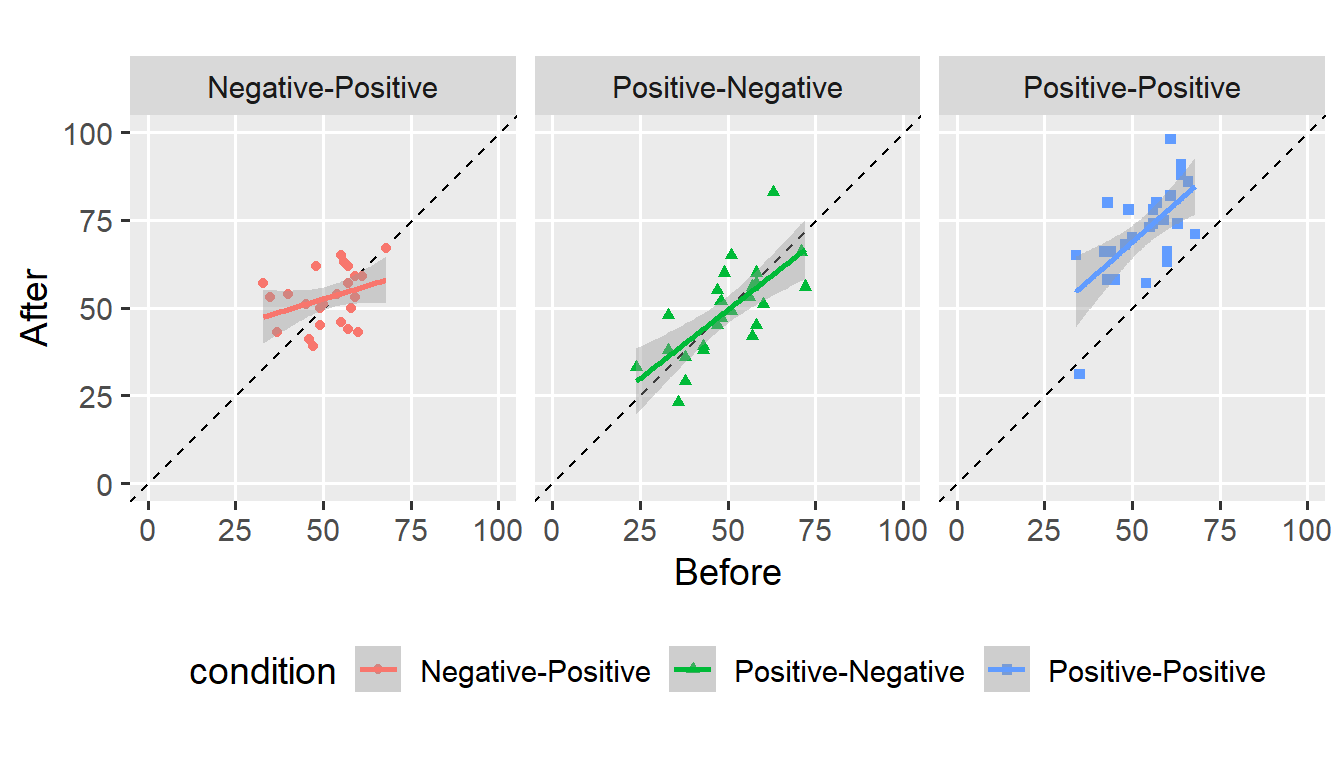Instead, we might remove the legend as it does not provide any additional information.

``fig + theme(legend.position = "none")```legend.position` is one of many arguments we can use to change how our plot looks. The `theme()` function is flexible and allows customising almost all elements that make up a plot. By default, `ggplot()` applies `theme_grey()` to create the now-familiar look. We can make this default explicit.

``````fig +
theme_grey(base_size = 14, base_line_size = 0.5) +
theme(legend.position = "none")``````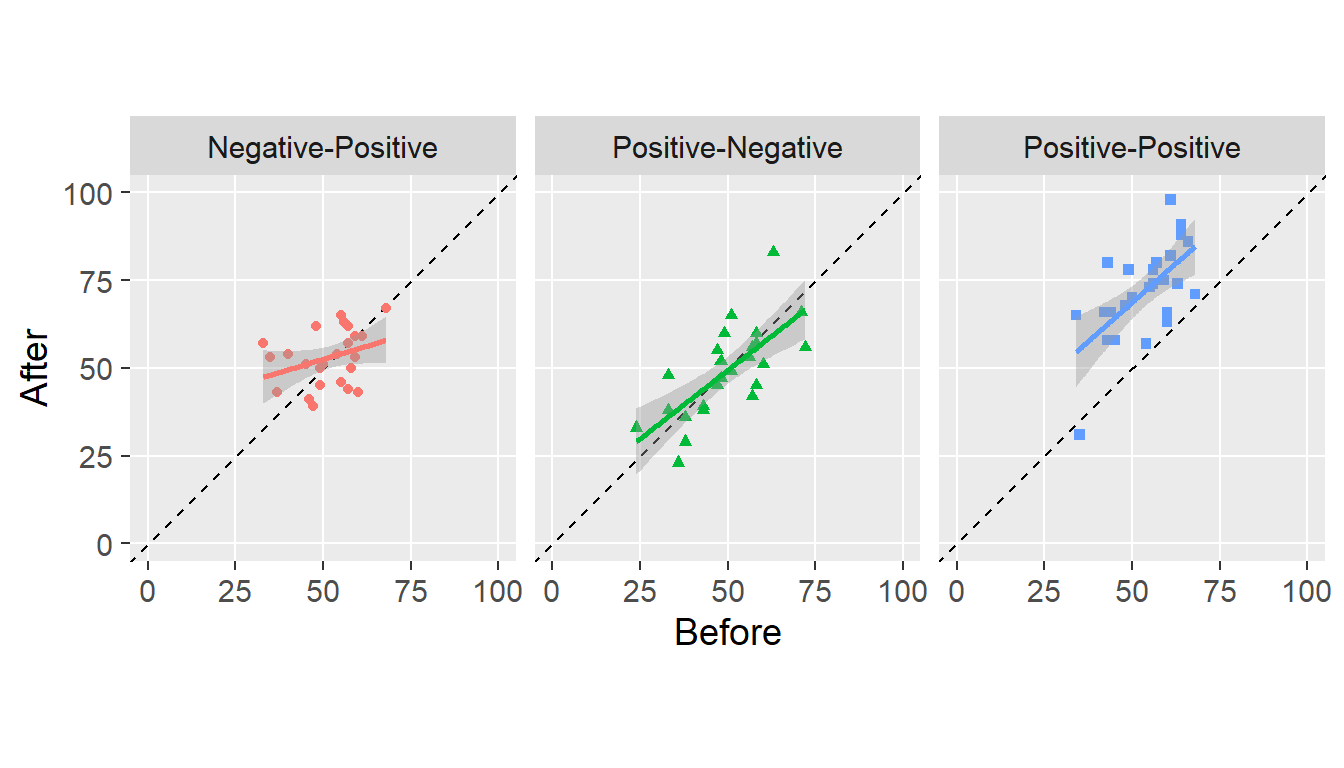We set the theme to `theme_grey()` and set the font size to 14 points.2 We can use other themes that come with `ggplot2`, for example, `theme_minimal()`.

``````fig +
theme_minimal(base_size = 14, base_line_size = 0.5) +
theme(legend.position = "none")``````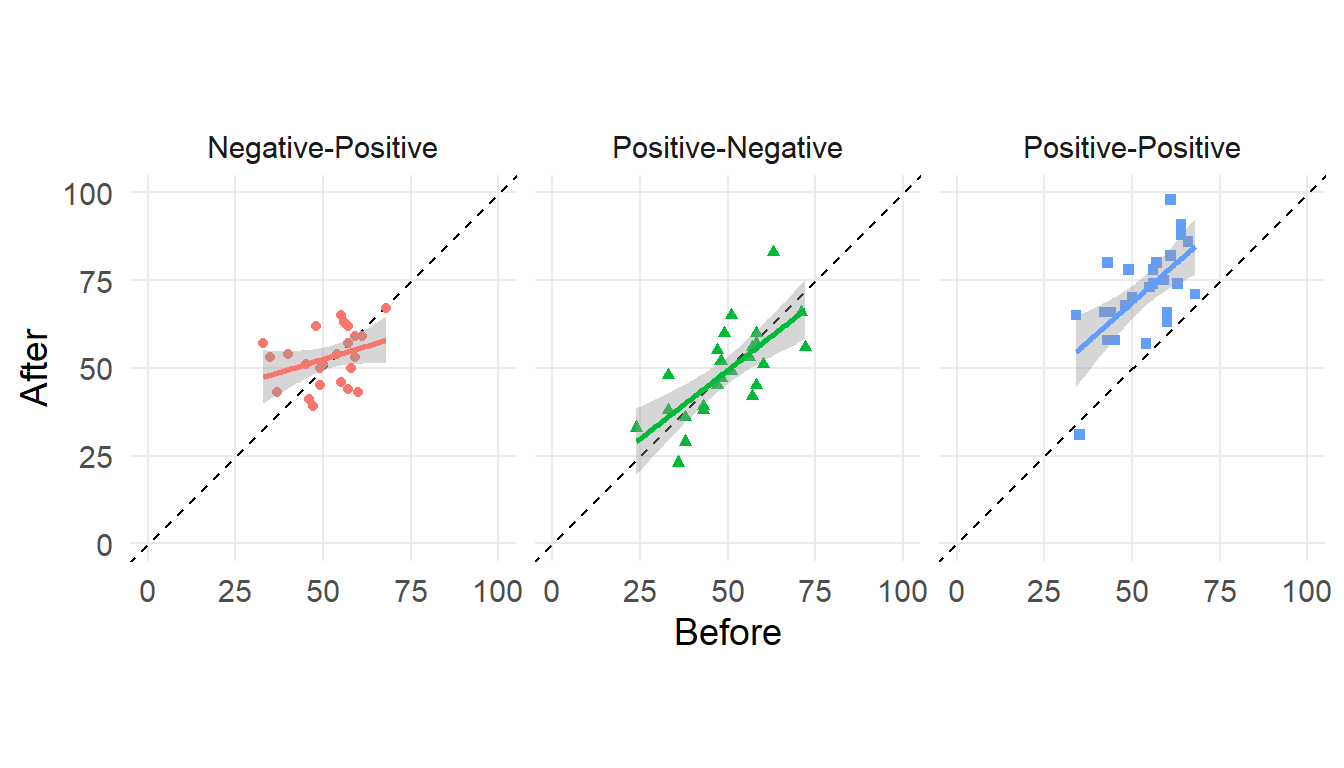What theme you choose is a matter of taste (and journal policy). I have grown to like the default theme and will use it for the next few examples.

Another thing we might want to change are labels. By default, `ggplot()` uses variable names to label the corresponding aesthetics. We can change these labels using the `labs()` function.

``````fig +
labs(
x = "Before",
y = "After"
) +
theme(legend.position = "none")``````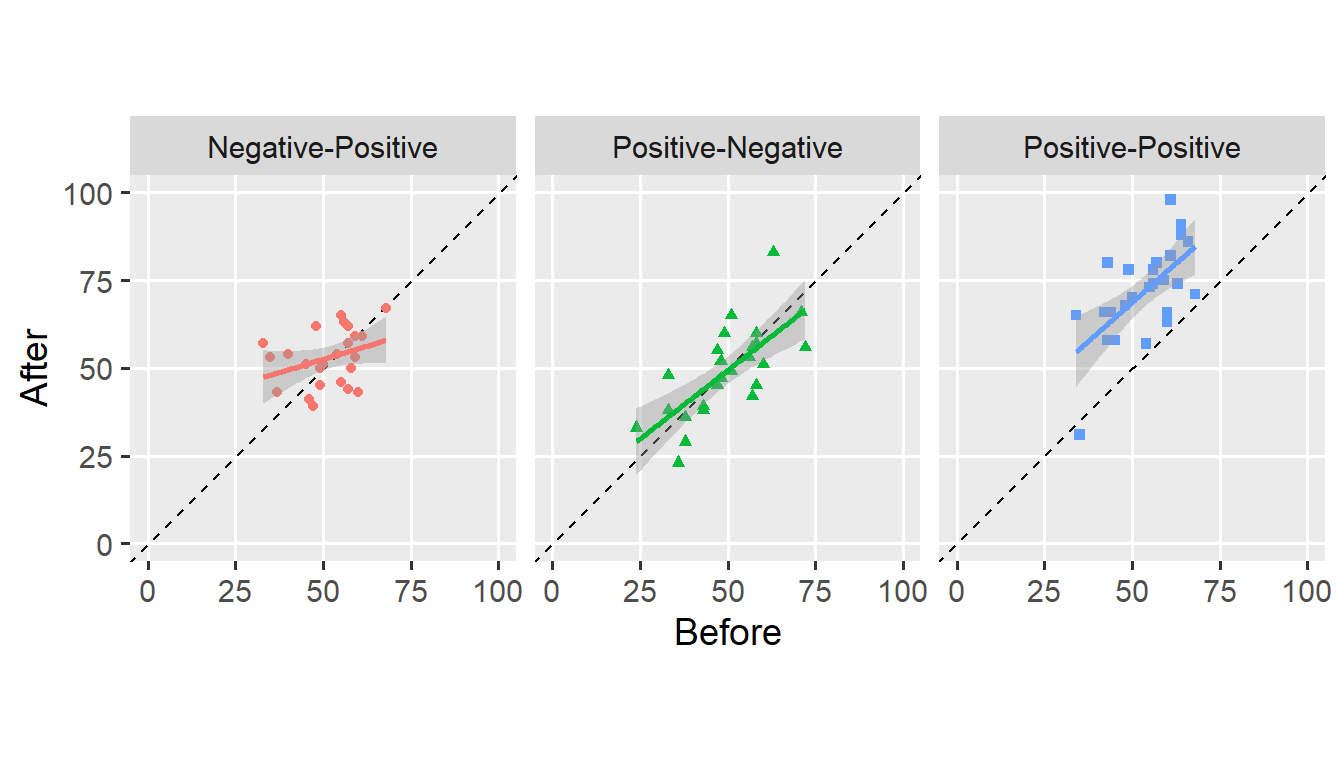In this case, I left the axis labels as they were. We can also use the `labs()` function to add a `title`, `subtitle`, and `caption` to the figure.

``````fig +
labs(
title = "Order effects in intergroup contact experiences",
subtitle = "Consecutive positive contact experiences improved attitudes,\nmixed experiences did not.",
caption = expression(italic("Reimer et al., 2018"))
) +
theme(legend.position = "none")``````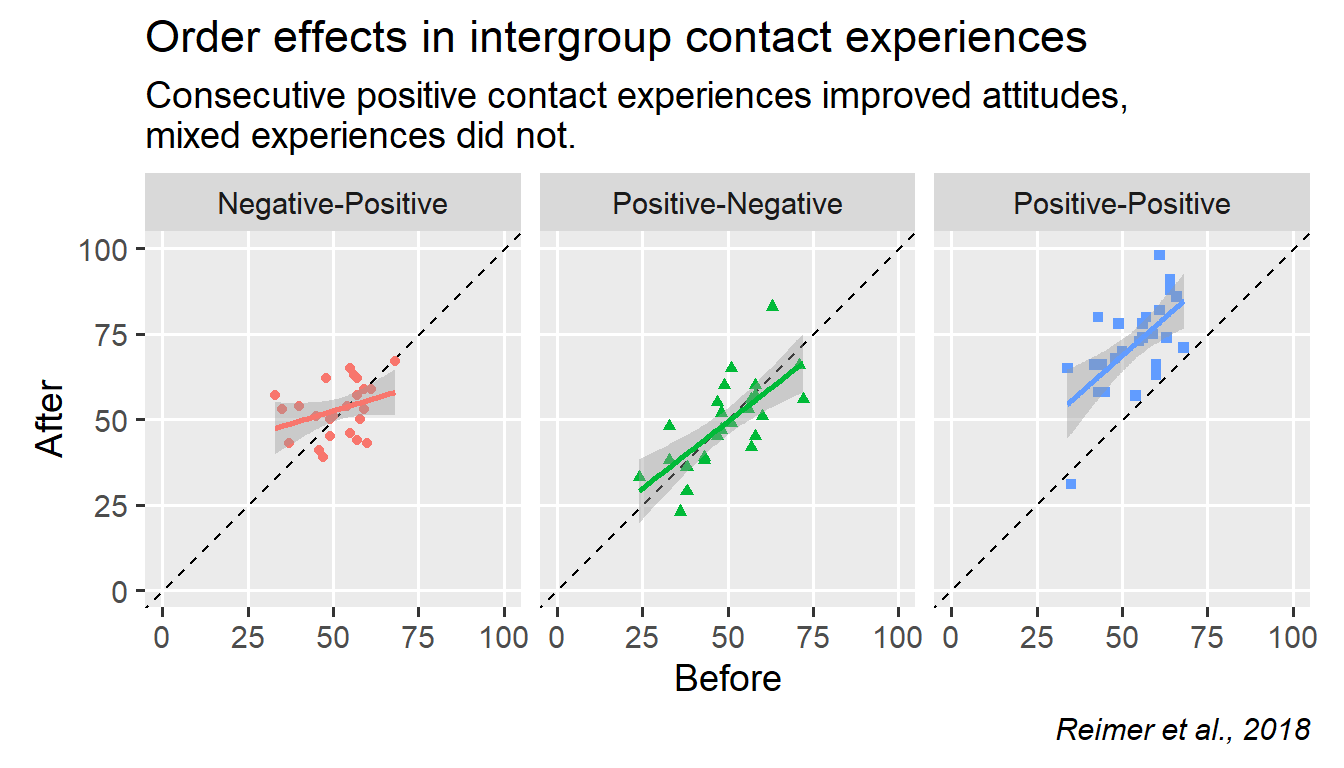Note that `\n` forces a line break in any character string (see `subtitle`). I think this would make a decent figure for publication, though others might prefer a more austere look.

We can install the `cowplot` package to achieve a more “serious” look.

``install.packages("cowplot")``

We call `theme_cowplot()` and add it to the plot.

``````fig +
cowplot::theme_cowplot(font_size = 14) +
theme(legend.position = "none")``````I don’t like the grey background behind the facet titles. We use the `theme()` function to change this. We also add a `background_grid()` to the theme.

``````fig +
cowplot::theme_cowplot(font_size = 14) +
cowplot::background_grid(major = "xy", minor = "none") +
theme(
legend.position = "none",
strip.background = element_blank()
)``````Keeping with the austere look, we also remove the `colour` and `shape` aesthetics.

``````ggplot(dw, aes(x = Before, y = After)) +
geom_abline(intercept = 0, slope = 1, linetype = "dashed") +
geom_point() +
geom_smooth(method = "lm", colour = "black") +
scale_x_continuous(limits = c(0, 100), minor_breaks = NULL) +
scale_y_continuous(limits = c(0, 100), minor_breaks = NULL) +
facet_grid(. ~ condition) +
coord_fixed(1) +
cowplot::theme_cowplot(font_size = 14) +
cowplot::background_grid(major = "xy", minor = "none") +
theme(
legend.position = "none",
strip.background = element_blank()
)``````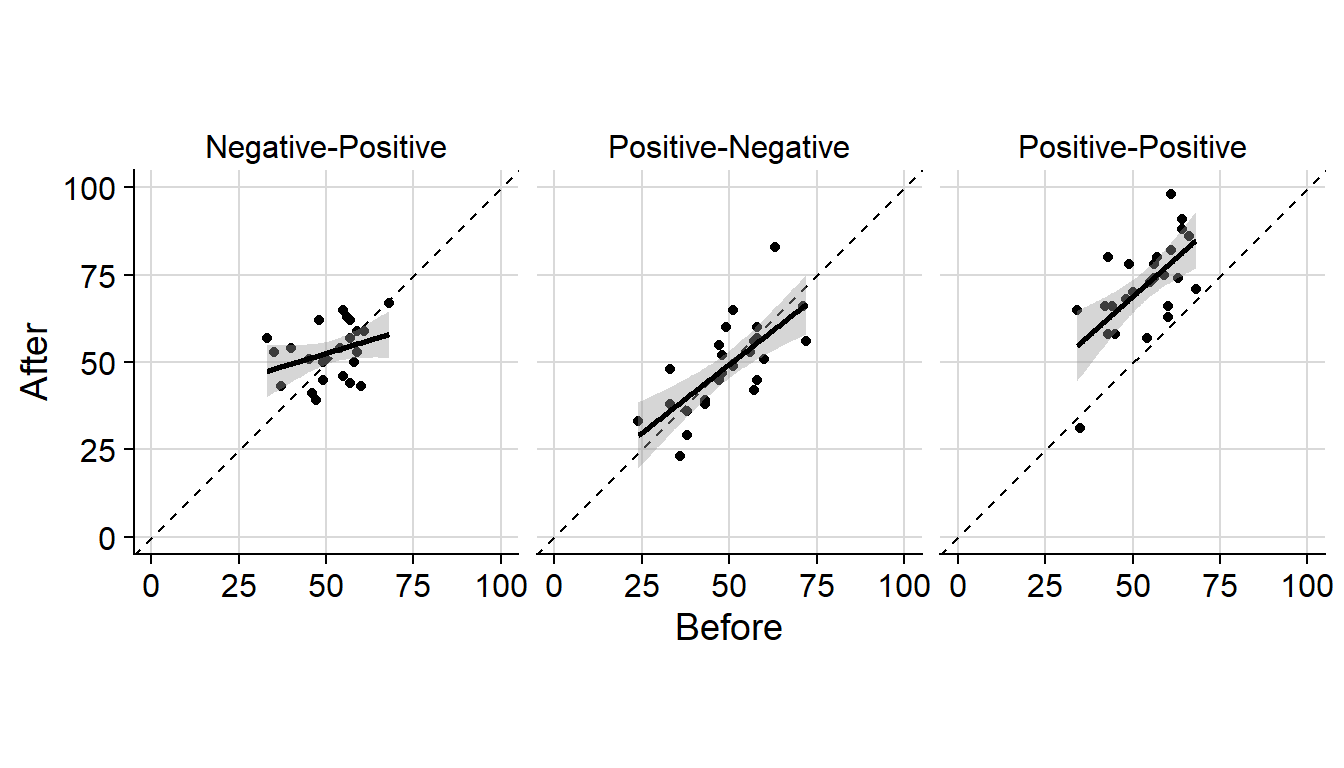All that’s left is to export the plot. We use the `ggsave()` function to export the plot.

``````ggsave("figure.pdf", height = 6, width = 14, units = "cm")
ggsave("figure.png", height = 6, width = 14, units = "cm",
dpi = 600, type = "cairo-png")``````

I prefer exporting figures to a vector format (such as .pdf). If you export a figure to a bitmap format (such as .png), you have to specify its resolution (as dots-per-inch). I recommend using `type = "cairo-png"` for smoother (anti-aliased) lines.

And that’s it for this post. You now understand how to prepare figures for publication. If you have a question or found a mistake, please comment on Twitter or send me an email.

Next week, we’ll take a brief look at annotations and text labels in `ggplot2`.

## Footnotes

1. The study I’m describing is real, though the data we’re using is simulated.↩︎

2. I’ve been using `theme_set(theme_grey(base_size = 14))` in every post to make figures legible online.↩︎